## Sunday, April 19, 2009

### Mathematics - Quadratic Equation (Part 1)Picture is obtained from http://foldspace.files.wordpress.com/2008/06/c4743r.jpg

The mathematics question is,
Find the value of k of the quadratic equationSuch that one of its roots is thrice the value of the other.

Solution:
Let α and β be the roots. We have,

Sum of roots:Product of roots:Given that,Substituting into eq. (1), we get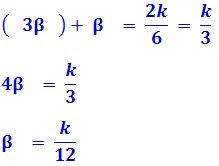Therefore the other root is,What to do next?
The roots,
α and β are found but in terms of k.
How to find the value of k?
Note that equation (2) is not yet used.Substituting α and β into eq. (2), we have,How to obtain the Quadratic Formula

In mathematics, a quadratic equation is a polynomial equation of the second degree. The general form is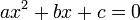The quadratic coefficients are the following:
a is the coefficient of x2
b is the coefficient of x
c is the constant coefficient (also called the free term or constant term).

The roots x or the quadratic formula can be derived by the method of completing the square. Dividing the quadratic equationby a (which is allowed because a is non-zero), gives: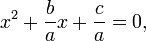orThe quadratic equation is now in a form to which the method of completing the square can be applied.
To "complete the square" add the constant,, to both sides,The left side is now a perfect square becauseSubstituting the left side with the perfect square we have,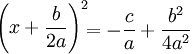The right side can be written as a single fraction, with common denominator 4a2. This gives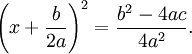Taking the square root of both sides yieldsIsolating x, gives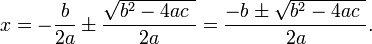This equation is known as the quadratic formula.

How to obtain Sum of roots and Product of roots

The general form is of a quadratic equation isDividing the quadratic equation by coefficient, a, we haveIf a quadratic equation has roots, α and β, the equation can be written asThat is,Comparing eq.(1) and eq.(2),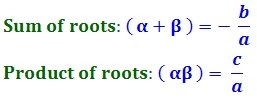Reference